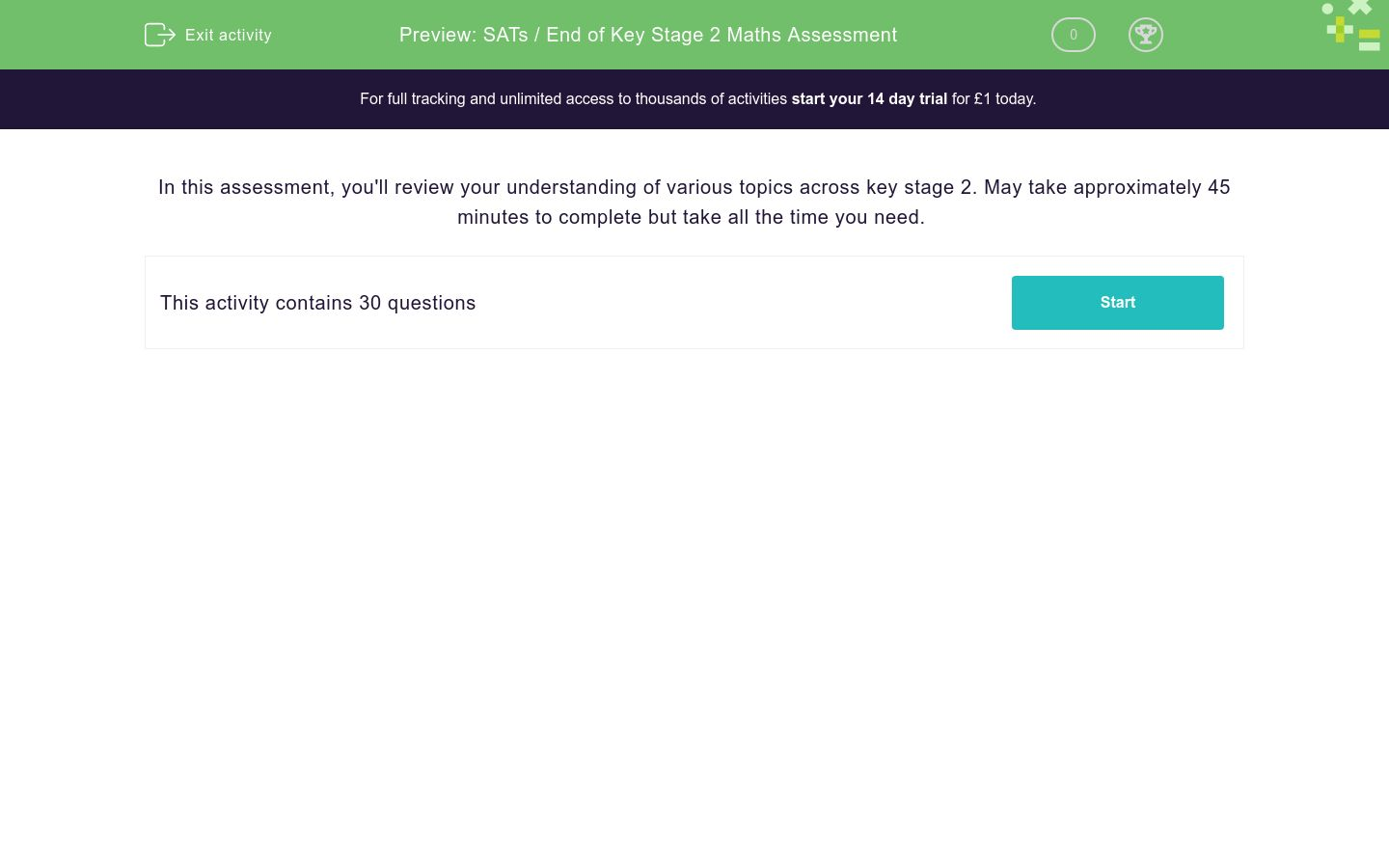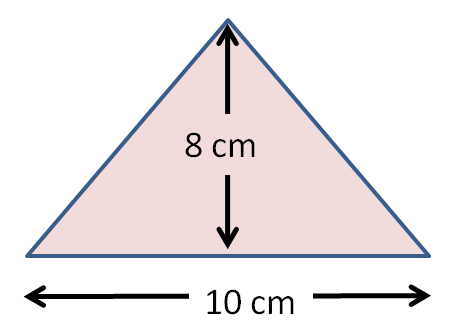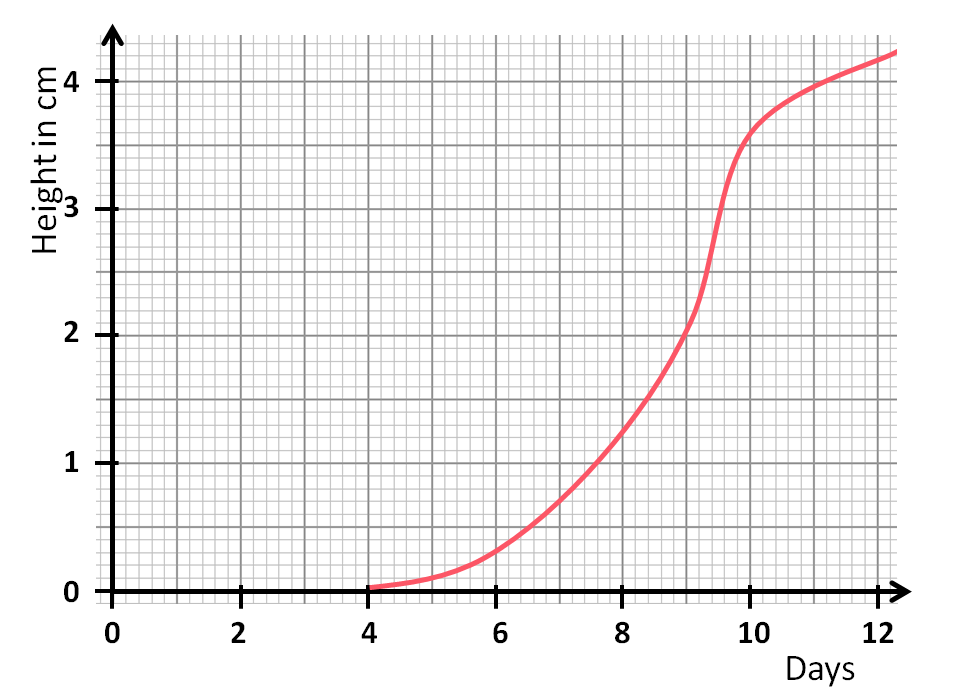SATs / End of Key Stage 2 Maths Assessment

In this assessment, students will review their comprehension of various topics across key stage 2. May take approximately 45 minutes to complete.Key stage:  KS 2

Curriculum topic:  End of Key Stage Assessments

Curriculum subtopic:  Starter Assessments

Difficulty level:QUESTION 1 of 10

In this assessment, you'll review your understanding of various topics across key stage 2. May take approximately 45 minutes to complete but take all the time you need.

Write this number using figures:

Fourteen thousand and twenty-two

How many ten thousands are there in the number:

4 521 637

1

2

3

4

5

6

7

When you double the number 156 000, which digit will be in the hundred thousands place?

Select all the prime numbers in this list.

1

2

3

4

5

9

13

25

29

33

Round to the nearest hundred thousand:

3 456 011

Work out:

25 + 30 ÷ 5 - 2

34 + 26

Use long multiplication to work out:

1616 × 34

Use long division to work out:

3396 ÷ 16

(If the answer has a remainder, write it like this...   321 r5)

Write down the number which is halfway between:

5400 and 10400

Choose all the fractions which are equivalent to the decimal:

0.375

375/100

375/1000

75/200

75/250

15/40

3/8

5/8

Work out a quarter of:

0.69

Convert the following percentage to a decimal:

99%

Work out the following, remembering to give your answer in its lowest terms, e.g. 7/10.

 3 - 7 5 20

Work out the following, remembering to reduce the answer to its lowest terms if possible:

 5 × 4 ÷ 1 9 5 18

Work out:

2.683 + 5.1

These two shapes are similar.

State the missing length in cm.

(Just write the number.)A camera normally costs £225.

How much will it cost in pounds in a 15% sale?

(Just write the number.)

I think of a number.

I double it and add 7.

I get 21.

Write this using algebra.

Use n for the number I think of.

7n + 2 = 21

7n - 2 = 21

2n + 7 = 21

2n - 7 = 21

To convert a temperature in Centigrade to one in Fahrenheit, we use this formula:

F = C × 9 ÷ 5 + 32

where

C is the temperature in degrees Centigrade or Celsius

F is the temperature in degrees Fahrenheit.

Use the formula to convert 40 degrees Centigrade to Fahrenheit.

(Just write the number.)

7a + 2b = -5

Which of these solutions satisfy the equation?

Tick all the solutions that fit.

a = -3, b = 8

a = -8, b = 25

a = -5, b = 15

a = 3, b = -13

a = 8, b = -25

Calculate the area of this triangle in cm2.

(Just write the number.)Which is further?

158 miles

248 km

Here is the net of a cuboid.

The squares have dimensions 5 cm by 5 cm.

The rectangles have dimensions 5 cm by 16 cm.

What is the volume of the cuboid in cm3?

(Just write the number.)On paper, draw a set of x-y coordinate axes like these.Follow these instructions to plot points and draw lines.

Name the shape that is formed.

Join (-2, -3) to (0, -3).

Join (0, -3) to (-1, -5).

Join (-1, -5) to (-2, -3).

isosceles triangle

right-angled triangle

square

rectangle

parallelogram

Look at the triangles on this coordinate grid.

On which triangle does Triangle G land when it is reflected in the y-axis?Look at the angles shown in the diagram below.

Work out the size of angle b.

Use this to write down the size of angle d.

Do not write down the size of angle b.

(Just write the number.)The children in a football team were asked to say their shoe size.

Work out the MODAL value or the MODE of this list.

5,  8,  7,  7,  6,  5,  6,  7,  6,  8,  7

This pie chart shows the holiday destinations of a class of children.What percentage of the class went to the UK?

90

25

4

¼

20

Look at this line graph, which shows the height of a tomato seedling, measured each day.

How many metres did the plant grow between day 7 and day 10?

(Just write the number.)• Question 1

Write this number using figures:

Fourteen thousand and twenty-two

14022
14 022
14,022
EDDIE SAYS
Fourteen thousand would be 14 000.
There are no hundreds.
Then we need 22 in the tens and units columns.
• Question 2

How many ten thousands are there in the number:

4 521 637

2
EDDIE SAYS
The 2 is in the ten thousands column.
• Question 3

When you double the number 156 000, which digit will be in the hundred thousands place?

3
EDDIE SAYS
156 000 × 2 = 312 000
• Question 4

Select all the prime numbers in this list.

2
3
5
13
29
EDDIE SAYS
Prime numbers only have two factors - the number itself and 1.
• Question 5

Round to the nearest hundred thousand:

3 456 011

3500000
3 500 000
EDDIE SAYS
3 456 011 is between 3 400 000 and 3 500 000 but closer to
3 500 000 because of the 5 in the ten thousands place.
• Question 6

Work out:

25 + 30 ÷ 5 - 2

29
EDDIE SAYS
Division takes priority over addition or subtraction.
30 ÷ 5 = 6
So this reads 25 + 6 - 2 = 29.
• Question 7

34 + 26

60
EDDIE SAYS
30 + 20 = 50
4 + 6 = 10
Add together 50 + 10 = 60.
• Question 8

Use long multiplication to work out:

1616 × 34

54944
54 944
EDDIE SAYS
1616 × 4 = 6464
1616 × 30 = 48 480
Add to get 54 944.
• Question 9

Use long division to work out:

3396 ÷ 16

(If the answer has a remainder, write it like this...   321 r5)

212 r4
212r4
EDDIE SAYS• Question 10

Write down the number which is halfway between:

5400 and 10400

7900
EDDIE SAYS
Difference is 5000.
Halve this to get 2500.
Add this to 5400.
• Question 11

Choose all the fractions which are equivalent to the decimal:

0.375

375/1000
75/200
15/40
3/8
EDDIE SAYS
By dividing top and bottom by 5, we see that 375/1000 reduces to 75/200, which reduces to 15/40, which reduces to 3/8.
• Question 12

Work out a quarter of:

0.69

0.1725
EDDIE SAYS
69 ÷ 2 = 34.5
34.5 ÷ 2 = 17.25
So 69 ÷ 4 = 17.25
0.69 ÷ 4 = 0.1725
• Question 13

Convert the following percentage to a decimal:

99%

0.99
EDDIE SAYS
99 ÷ 100 = 0.99
• Question 14

Work out the following, remembering to give your answer in its lowest terms, e.g. 7/10.

 3 - 7 5 20
1/4
EDDIE SAYS
The lowest common multiple of 5 and 20 is 20.
This gives 12/20 - 7/20 = 5/20 = 1/4.
• Question 15

Work out the following, remembering to reduce the answer to its lowest terms if possible:

 5 × 4 ÷ 1 9 5 18
8
EDDIE SAYS
Turn 1/18 upside down.
Reduce the 5s.
Reduce the 18 and 9.
Multiply the tops and multiply the bottoms, giving 8/1.
• Question 16

Work out:

2.683 + 5.1

7.783
EDDIE SAYS
Add units to units and tenths to tenths.
• Question 17

These two shapes are similar.

State the missing length in cm.

(Just write the number.)21
EDDIE SAYS
Ratio is 1:3, so missing length is 3 × 7 = 21.
• Question 18

A camera normally costs £225.

How much will it cost in pounds in a 15% sale?

(Just write the number.)

191.25
EDDIE SAYS
10% of 225 = 22.50
5% of 225 = 11.25
15% of 225 = 22.50 + 11.25 = 33.75
Work out 225 - 33.75.
• Question 19

I think of a number.

I double it and add 7.

I get 21.

Write this using algebra.

Use n for the number I think of.

2n + 7 = 21
EDDIE SAYS
Double n and add 7 to get 21.
• Question 20

To convert a temperature in Centigrade to one in Fahrenheit, we use this formula:

F = C × 9 ÷ 5 + 32

where

C is the temperature in degrees Centigrade or Celsius

F is the temperature in degrees Fahrenheit.

Use the formula to convert 40 degrees Centigrade to Fahrenheit.

(Just write the number.)

104
EDDIE SAYS
C = 40
F = 40 × 9 ÷ 5 + 32 = 360 ÷ 5 + 32 = 72 + 32 = 104
• Question 21

7a + 2b = -5

Which of these solutions satisfy the equation?

Tick all the solutions that fit.

a = -3, b = 8
a = -5, b = 15
a = 3, b = -13
EDDIE SAYS
7 × -3 + 2 × 8 = -21 + 16 = -5
7 × -8 + 2 × 25 = -56 + 50 = -6
7 × -5 + 2 × 15 = -35 + 30 = -5
7 × 3 + 2 × -13 = 21 + -26 = -5
7 × 8 + 2 × -25 = 56 - 50 = 6
• Question 22

Calculate the area of this triangle in cm2.

(Just write the number.)40
EDDIE SAYS
Base length = 10 cm
Perpendicular height = 8 cm
Area = 10 × 8 ÷ 2 = 80 ÷ 2 = 40 cm2
• Question 23

Which is further?

248 km
EDDIE SAYS
248 km = 248 ÷ 8 × 5 = 32 × 5 = 160 miles
• Question 24

Here is the net of a cuboid.

The squares have dimensions 5 cm by 5 cm.

The rectangles have dimensions 5 cm by 16 cm.

What is the volume of the cuboid in cm3?

(Just write the number.)400
EDDIE SAYS
Dimensions of cuboid will be 5 cm by 5 cm by 16 cm.
Volume = 5 × 5 × 16 = 5 × 80 = 400 cm3
• Question 25

On paper, draw a set of x-y coordinate axes like these.Follow these instructions to plot points and draw lines.

Name the shape that is formed.

Join (-2, -3) to (0, -3).

Join (0, -3) to (-1, -5).

Join (-1, -5) to (-2, -3).

isosceles triangle
EDDIE SAYS• Question 26

Look at the triangles on this coordinate grid.

On which triangle does Triangle G land when it is reflected in the y-axis?F
EDDIE SAYS
G flips over the y-axis to land on F.
• Question 27

Look at the angles shown in the diagram below.

Work out the size of angle b.

Use this to write down the size of angle d.

Do not write down the size of angle b.

(Just write the number.)62
EDDIE SAYS
Angle b = 180 - 107 = 73°
Angles in a triangle add to 180°.
d = 180 - 73 - 45 = 180 - 117 = 62°
• Question 28

The children in a football team were asked to say their shoe size.

Work out the MODAL value or the MODE of this list.

5,  8,  7,  7,  6,  5,  6,  7,  6,  8,  7

7
EDDIE SAYS
7 is the one that is listed the most times.
• Question 29

This pie chart shows the holiday destinations of a class of children.What percentage of the class went to the UK?

25
EDDIE SAYS
90° of the circle is ¼ of the circle.
¼ = 25%
• Question 30

Look at this line graph, which shows the height of a tomato seedling, measured each day.

How many metres did the plant grow between day 7 and day 10?

(Just write the number.)0.029
EDDIE SAYS
At day 7, the height was 0.7 cm = 7 mm.
At day 10, the height was 3.6 cm = 36 mm.
36 - 7 = 29 mm = 0.029 m
---- OR ----

Sign up for a £1 trial so you can track and measure your child's progress on this activity.

What is EdPlace?

We're your National Curriculum aligned online education content provider helping each child succeed in English, maths and science from year 1 to GCSE. With an EdPlace account you’ll be able to track and measure progress, helping each child achieve their best. We build confidence and attainment by personalising each child’s learning at a level that suits them.

Get startedStart your £1 trial today.
Subscribe from £10/month.